Courses

# The Kinetic Theory Of Gases - Gaseous Chemistry Notes | EduRev

## Chemistry : The Kinetic Theory Of Gases - Gaseous Chemistry Notes | EduRev

The document The Kinetic Theory Of Gases - Gaseous Chemistry Notes | EduRev is a part of the Chemistry Course Physical Chemistry.
All you need of Chemistry at this link: Chemistry

The Kinetic Theory of Gases

In order to derive the theoretical aspect of the various gas laws based on simple experiment facts, Maxwell proposed the following postulates under the heading of kinetic theory of gases:

The postulates of kinetic theory of gas are

• Each gas is made up of a large number of small (tiny( particles known as molecules.

• The volume of a molecule is so small that it may be neglected in comparison to total volume of gas.

• The molecules are never in stationary state but they are believed to be in chaotic (random) motion. They travel in straight line in all possible directions with altogether different but constant velocities. The direction of motion is changed by the collision with container or with the other molecules.

• The collision between molecules is perfectly elastic i.e., there is no change in the energies of the molecules after collision.

• The effect of gravity on molecular motion is negligible.

• The kinetic energy of the gases depends on the temperature.

• The pressure of the gas arises due to collision due to collision of molecules with the walls of the container.

The Kinetic Equation: Maxwell also derived an equation on the basis of above assumptions as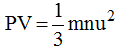where

P = Pressure of gas

V = Volume of gas

m = mass of one molecules of gas

n = number of molecules of gas

u = root mean square velocity of molecules

For 1 mole n = N (Avogadro number)

m ×  N = Molecular mass M.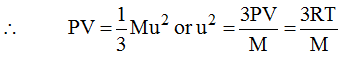or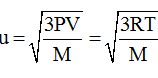Distribution of Molecular Velocities

Maxwell and Boltzmann proposed that gas molecules are always in rapid random motion colliding with each other and with the walls of container. Due to such collisions, their velocities always changes. A fraction of molecules have a particular molecular velocity at a time. James Clark Maxwell calculated the distribution of velocity among fractions of total number of molecules, on the basis of probability.

The distribution of velocities of different gas molecules may be shown by the following curve.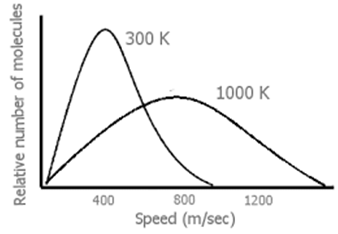From the curve it may be concluded that

(i)         Only a small fraction of molecules have either very low or very high velocity.

(ii)        Curve becomes flat when temperature is raised i.e. distribution around average velocity becomes wider. Average molecular velocity increases with rise in temperature.

(iii)       Most of the molecules have velocity close to most probable velocity represented by the top of curve.

(iv)       At higher temperature greater number of molecules have high velocity, while few molecules have lower velocity.

Average Velocity: As per kinetic theory of gases, each molecule is moving with altogether different velocity. Let ‘n’ molecules be present in a given mass of gas, each one moving with velocity u1, u2, u3…….., un.

The average velocity or Uav = average of all such velocity terms.

Average Velocity =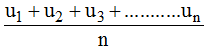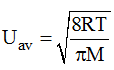Root Mean Square Velocity: Maxwell proposed the term Urms as the square root of means of square of all such velocities.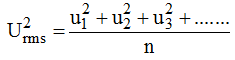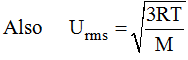Most probable velocity:  It is the velocity possessed by maximum number of molecules.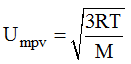Furthermore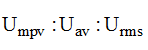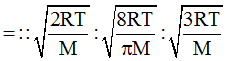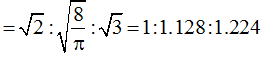Also Uav = Urms × 0.9213

Kinetic Energy of Gas:  As per kinetic equation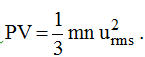.

For 1 mole  m × n = Molecular Mass (M)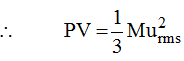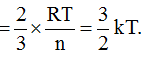Where k is the Boltzmann constant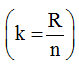Kinetic Energy of gas sample:

(i)         Average kinetic energy of a single molecule =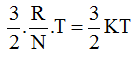K = boltzmann constant = 1.38 × 10–23 J/deg

(ii)         Total kinetic Energy for one mole of gas =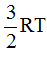(iii)       Kinetic Energy for n mol of gas =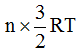Deviations from Ideal Behaviour

An ideal gas is one which obeys the gas laws of the gas equation PV = RT at all pressure and temperatures. However no gas in nature is ideal. Almost all gases show significant deviations from the ideal behaviour. Thus the gases H2, N2 and CO2 which fail to obey the ideal-gas equation are termed as non-ideal or real gases.

Compressibility Factor: The extent to which a real gas departs from the ideal behaviour may be depicted in terms of a new function called the compressibility factor, denoted by Z. It is defined as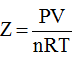The deviations from ideality may be shown by a plot of the compressibility factor Z, against P.

For an ideal gas, Z = 1 and it is independent of temperature and pressure.

The deviations from ideal behaviour of a real gas will be determined by the value of Z being greater or less than 1.

The difference between unity and the value of the compressibility factor of a gas is a measure of the degree of non ideality of the gas.

For a real gas, the deviations from ideal behaviour depends on:

(i) pressure; and        (ii) temperature.

This will be illustrated by examining the compressibility curves of some gases discussed below with the variation of pressure and temperature.

Effect of Pressure Variation on Deviations: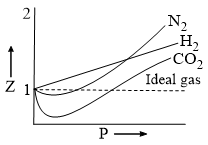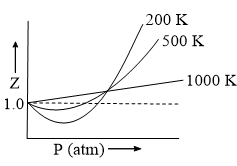From the above curves we can conclude that:

• At low pressure and fairly high temperatures, real gases show nearly ideal behaviour and the ideal-gas equation is obeyed.

• At low temperatures and sufficiently high pressure, a real gas deviates significantly from ideality and the ideal-gas equation is no longer valid.

• The closer the gas is to the liquefication point, the larger will be the deviation from the ideal behaviour.

Greater is the departure Z from unity, more is the deviation from ideal behaviour.

(i)               When Z < 1, this implies that gas is more compressible.

(ii)              When Z > 1, this means that gas is less compressible.

(iii)             When Z = 1, the gas is idea.

Vander Waals Equation of State for Real Gas: the equation of state generated by Vander Waals in 1873 reproduces the observed behaviour with moderate accuracy. For a node of gas, the Vander Waals equation is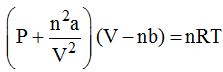Where a and b are constants characteristic of a gas.

Volume Correction:  We know that for an ideal gas P × V = nRT. Now in a real gas the molecular volume cannot be ignored and therefore let us assume that ‘b’ is the volume excluded (out of the volume of container) for the moving gas molecules per mole of a gas. Therefore due to n moles of a gas the volume excluded would be nb.

∴ a real gas in a container of volume V has only available volume of (V – nb) and this can be through of, as an ideal gas in a container of volume (V – nb)

Pressure Correction: Let us assume that the real gas exerts a pressure P. The molecules that exert the force on the container will get attracted by molecules of the immediate layer which are not assumed to be exerting pressure.

It can be seen that pressure the real gas exerts would be less than the pressure an ideal gas would have exerted. Therefore if a real gas exerts a pressure P, then an ideal gas would exert a pressure equal to P + p(p is the pressure lost by the gas molecules due to attractions). This small pressure p would be directly proportional to the extent of attraction between the molecules which are hitting the container wall and the molecules which are attracting these.

Therefore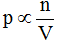(concentration of molecules which are hitting the container’s wall)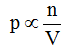(concentration of molecules which are attracting these molecules)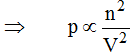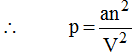Where a is the constant of proportionality which depends on the nature of gas. Higher value of ‘a’ reflects the increased attraction between gas molecules.

The Vander Waals constant b (the excluded volume) is actually 4 times the volume of a single molecule. i.e., b = 4 NAV where NA → Avogadro number.

b = 4 × 6.023 × 1023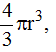where r is the radius of a molecule.

The constant a and b: Vander Waals constant for attraction (A) and volume (B) are characteristic for a given gas. Some salient features of ‘a’ and ‘b’ are:

1. For a given gas Vander Waals constant of attraction ‘a’ is always greater than Vander Waals constant of volume (B).
2. The gas having higher value of ‘a’ can be liquefied easily and therefore H2 and He are not liquefied easily.
3. The units of a = litre2 atm mole–2 and that b = litre mole–1
4. The numerical values of a and b are in the order of 10–1 to 10–2 to 10–4 respectively.
5. Higher is the value of ‘a’ for a given gas, easier is the liquification.

Explanation of deviation by Van der Waals equation

(i)      At lower pressure:  ‘V’ is large and ‘b’ is negligible in comparison with V.

Then Vander Waals equation reduces to: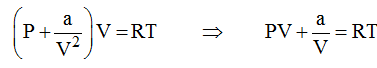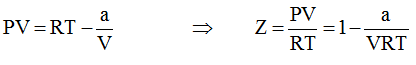Or PV < RT at low pressure (below Boyle temperature)

This accounts for the dip in PV vs P isotherm at low pressure.

(ii)    At fairly high pressure: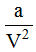may be neglected in comparison with P.

The Vander Waals equation becomes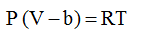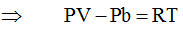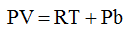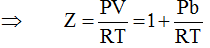Or PV > RT at higher pressure (above Boyle temperature)

(iii)  At very low pressure: V becomes so large that both b and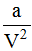become negligible and the Vander Waal equation reduces to PV = RT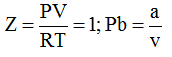At extremely low pressure (at Boyle temperature)

This shows why gases approach ideal behaviour at very low pressure.

(iv)   Hydrogen and Helium: These are two lightest gases known. Their molecules have very small masses the attractive forces between such molecules will be extensively small. So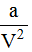is negligible even at ordinary temperature. Thus PV > RT.

Dieterici Equation:

P(V – nb) nRT ea/VRT

Berthelot Equation: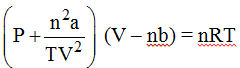Viral Equation of State For 1 Mole of Gas: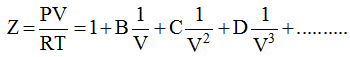B = second virial co-efficient, temperature dependent =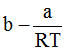C = third viral co-efficient, temperature, dependent = 1

Critical phenomenon & Liquefaction of gases

The phenomena of converting a gas into liquid is known as liquefaction. The liquefaction of gas is achieved by controlling P and T as follows:

1.  Increasing pressure: As increase in results in an increase in attraction among molecules.

2.  Decreasing temperature: A decrease in temperature results in decrease in kinetic energy of molecules.

Critical temperature (Tc): It is defined as the characteristic temperature for a given gas below which a continuous increase in pressure will bring liquefaction of gas and above which no liquefaction is noticed although pressure may be increased e.g. Tc for CO2 is 31.2°C.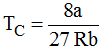Critical pressure (Pc): It is defined as the minimum pressure applied on 1 mole of gas placed at critical temperature, to just liquefy the gas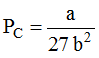Critical Volume (Vc): The volume occupied by 1 mole of gas placed at critical conditions.

VC = 3b  (i.e. P = Pc and T = Tc)

Collision parameters

• Mean free path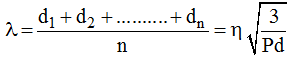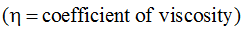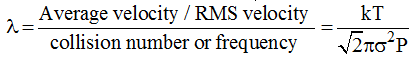K = Boltzman constant; s = collision diameter.

• Collision frequency (z): number of collision taking place per second per unit volume.

• Collision diameter: Closest distance between the centre of two molecule which are participating in collision.

• Relative Humidity (RH):

At a given temperature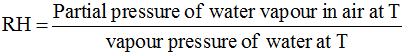• Loschmidth Number

The number of molecules present in 1 cc of gas (or) vapour at STP.

Value: 2.617 × 1019 1 cc

Offer running on EduRev: Apply code STAYHOME200 to get INR 200 off on our premium plan EduRev Infinity!

## Physical Chemistry

84 videos|106 docs|31 tests

,

,

,

,

,

,

,

,

,

,

,

,

,

,

,

,

,

,

,

,

,

;